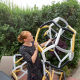# Bonus #MathArtChallenge: Y-Labyrinths

The Challenge: Using a hexagonal grid, create a labyrinth using “Y” shapes.

Materials needed: hexagonal/isometric grid (you can print from the internet or obtain from a compass), writing utensil, randomizer (die, coin, etc.)
Math Concepts you could explore with this challenge: 2D, combinations & permutations, counting, isometric, probability, randomness

Depending on how you use this activity, you may engage with different mathematical standards. I’ve listed possible connected math content above. Here are a few suggestions for how you might integrate the 8 mathematical practices. Feel free to add your own suggestions in the comments!

4.) Model with mathematics. By randomly assigning each block as a regular Y or an upside down Y, you create or end various paths. What combinations lead to a closed path? What combinations yield a long one?

7.) Look for and make use of structure. If you build this from an isometric grid, what do you notice about how the Y shapes interact with the hexagons? How can you describe this transformation?## Author: Ms. P

Math Teacher in Minneapolis, MN.

Posted on